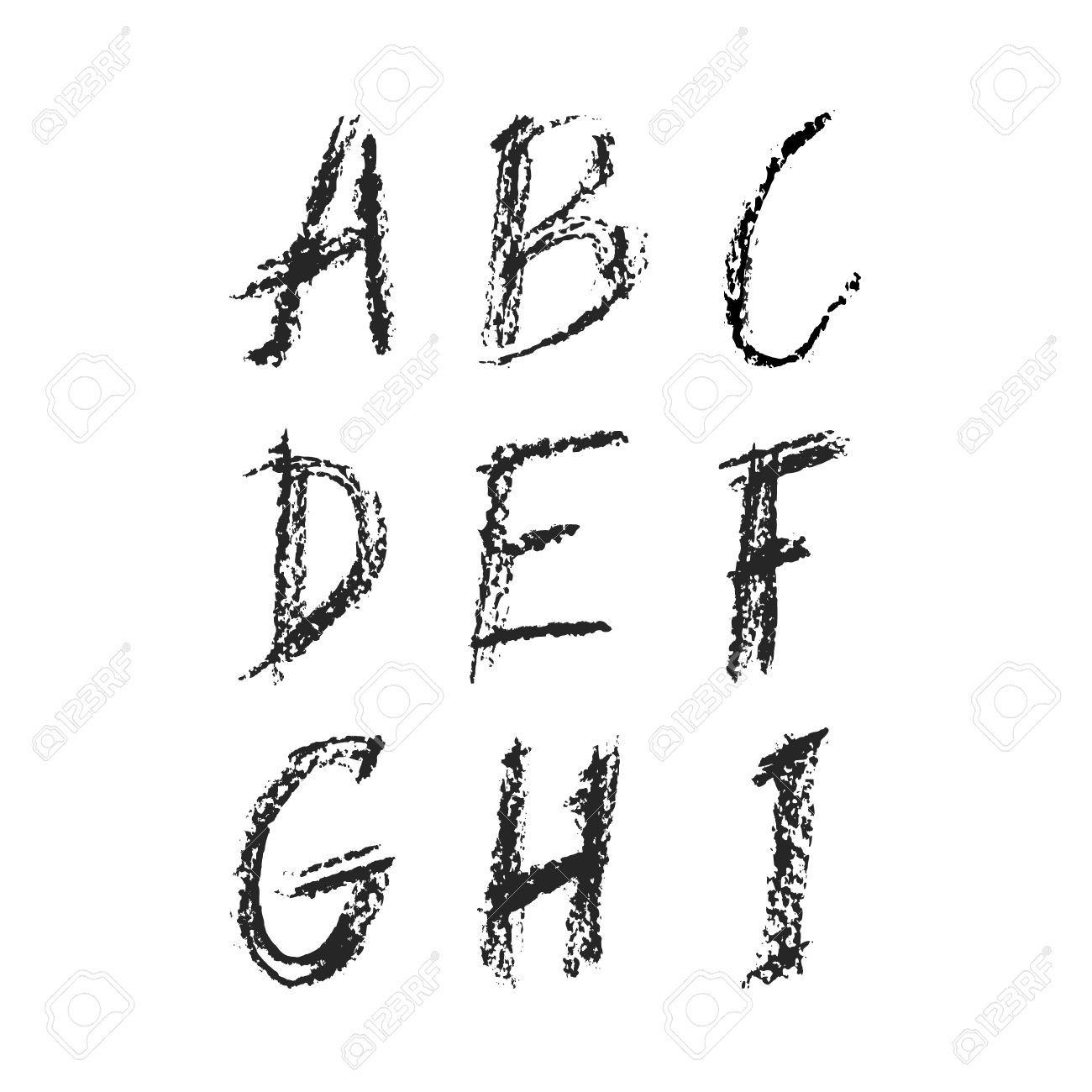# Logical Numbers

Logic Level 3All digits 1 to 9 are used in place of the letters A through I such that

• $A+B+C$ > $D+E+F$ > $G+H+I$

• $E$ is a prime factor of $G$

• $F$ > $A$

• $B+G= H$

• $I$ is not $1$

Which letter represents which number?

Concatenate the answer as the nine-digit integer $\overline{ABCDEFGHI}$.

×